# [Fixed!] Formula Result Showing 0 in Excel (3 Solutions)

In this tutorial, we will demonstrate how to fix the problem of the formula result showing 0 in excel. While working in Microsoft Excel we might need to add some values with a formula. But instead of showing the calculated value, the formula returns 0. You can face this problem for various reasons. Throughout this article, we will try to make your concept clear about how to fix this problem.

You can download the practice workbook from here.

## 3 Effective Solutions to Formula Result Showing 0 in Excel

We will discuss 3 effective solutions to fix the problem of the formula result showing 0 in excel. To illustrate the solutions to you, we will use the same dataset for ‘solution-1’ and ‘solution-2’ and slightly modified dataset for ‘solution-3’.

### 1. Fix Formula Result Showing 0 by Converting Text to Number

To fix the problem of the formula result showing 0 in excel first and foremost, we will check the data in our dataset that we will input into the formula. Sometimes the numbers in data are stored in text format. So, the formula returns 0 instead of the actual result. To solve this problem we will convert text to numbers.

#### 1.1 Convert Text to Number with Mouse Click

In this method, we will convert text to numbers with a mouse click to fix the problem of the formula result showing 0 in excel. In the following screenshot, we have a dataset of a bookstore. The dataset consists of the names of some books and the available quantity of those books in that book store. Suppose we want to calculate the number of total books in cell C10.Follow the below steps:

• Input the formula in cell C10 to calculate the total quantity:
`=SUM(C5:C9)`

####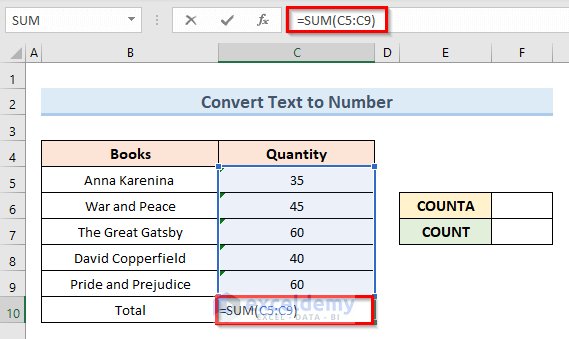• Press Enter and we can see that the formula returns 0. This is the problem that we need to fix.

####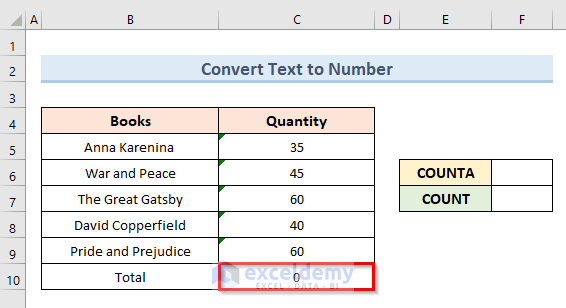• Before starting we want you to notice some points. In cell F6 insert the following formula:
`=COUNTA(C5:C9)`

####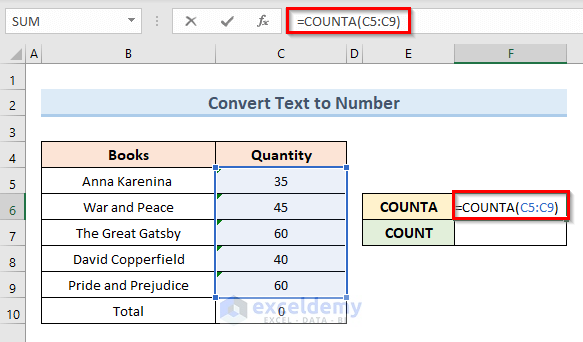• If you press Enter after inserting the formula you get the result because the COUNTA function counts the number of non-blank cells. It has no relation to the cell value.

####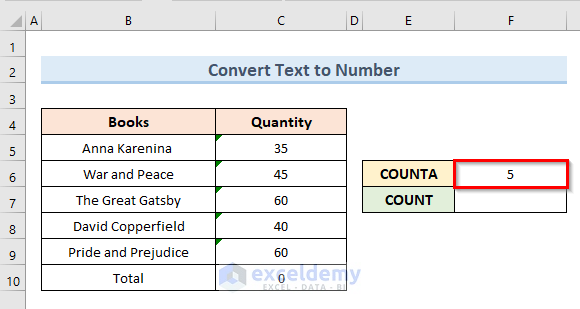• Again insert the following formula in cell F7:
`=COUNT(C5:C9)`
• Press Enter. Here the formula returns 0 because the COUNT function counts the number of cells that contain numbers not text.

####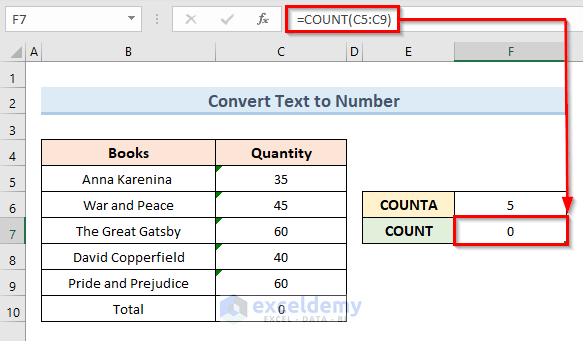• To understand the reason for showing 0 select cell C5 and look at the formula bar. We can see the number but there is an apostrophe that indicates the number is in text format. That is why we get an error while using formulas.

####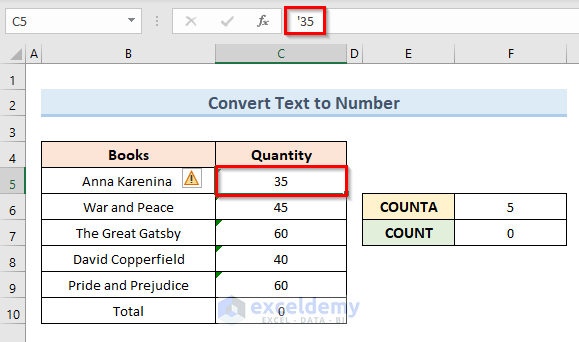Let’s see the steps to fix this problem.

STEPS:

• Firstly, select cell (C5:C9).

####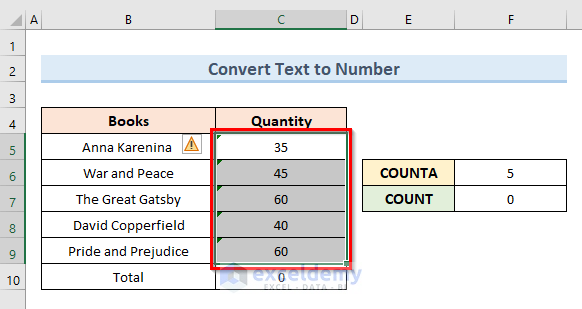• Secondly, click on the exclamation A drop-down menu will appear.
• Thirdly, select the option ‘Convert to Number’ from the drop-down menu.

####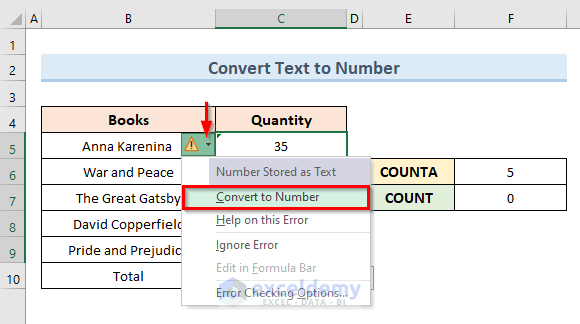• Lastly, we can see that the formula result is not showing 0 anymore in cells C10 and F7. Since we have converted the format of values in cell (C5:C9) from text to number, the formula in cell C10 can give the result now.

####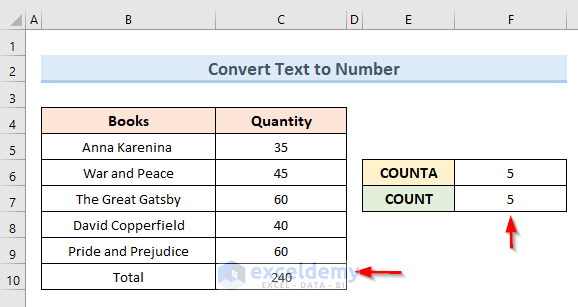Read More: [Fixed!] Formula Not Working and Showing as Text in Excel

#### 1.2 Use ‘Paste Special’ Option to Convert Text to Number

Another method to fix the problem of the formula result showing 0 in excel is to use the ‘Paste Special’ option. To illustrate this method we will use the same dataset that we used in the previous method.So, let’s see the steps to use the ‘Paste Special’ option in this method.

STEPS:

• First, select any cell outside the data range and click on Copy.
• Next, select the cell range (C5:C9).
• Then, go to Home > Paste > Paste Special• The above actions will open a new dialogue box named ‘Paste Special’.
• After that, check the option Add under the Operation section and click on OK.• Finally, we can see the output of the formula in cell C10.Read More: How to Show All Formulas in Excel (4 Easy & Quick Methods)

#### 1.3 Apply VBA Code to Convert Text to Number to fix formula result showing 0 in excel

If you are an advanced excel user and want to perform tasks more quickly you can use VBA code to solve the above problem. In this method, we will apply a VBA code to fix the problem of the formula result showing 0 in excel more quickly.So, let’s see the steps to apply VBA code in this method.

STEPS:

• In the beginning, select cell (C5:C9).
• Next, right-click on the active sheet.
• Then, select the option ‘View Code’.• The above action will open a blank VBA module.
• Insert the following code in that module:
``````Sub TextToNumber()
Dim rg As Range
On Error Resume Next
Set rg = Selection _
.SpecialCells(xlCellTypeConstants, 23)
On Error GoTo ErrorHandle
If Not rg Is Nothing Then
Cells.SpecialCells(xlCellTypeLastCell) _
.Offset(0, 1).Copy
rg.PasteSpecial Paste:=xlPasteValues, _
Else
MsgBox "Unable to find Constants in selection"
End If
Handler_Exit:
Application.CutCopyMode = False
Set rg = Nothing
Exit Sub
ErrorHandle:
MsgBox "Unable to convert text to numbers"
Resume Handler_Exit
End Sub``````
• Now, click on the Run button or press the F5 key to run the code.• Finally, the above code fixes the problem of the formula result showing 0 in excel. So, we get the summation in cell C10.Read More: How to Show Value Instead of Formula in Excel (7 Methods)

### 2. Fix Formula Result Is Showing 0 in Excel Using ‘Text to Column’ Option

The use of the ‘Text to Column’ option from the Data tab is another effective way to fix the problem formula result showing 0 in excel. In the following dataset in cell C10, we will bring results instead of 0.Let’s see the steps to perform this action.

STEPS:

• First, select cell (C5:C9).
• Next, go to the Data Select the option ‘Text to Column’ from the ‘Data Tools’ section.• Then, a new dialogue box will appear. Check the option Delimited from the file type options and click on the Finish button.• In the end, the above action returns the result of the formula in cell C10.Read More: [Fixed!] Why Formula Is Not Working in Excel (15 Reasons with Solutions)

### 3. Remove Hidden Characters When Formula Result Showing 0 in Excel

Another reason for the formula result showing 0 in excel is the presence of hidden characters in the formula range. Sometimes we copy or download a dataset from another source. That dataset might contain hidden characters. To get the proper result we need to remove those hidden characters.

#### 3.1 Fix Formula Result Showing 0 Removing Hidden Characters Using Character Code

The hidden character can be a non-breaking space. In Microsoft Excel, the character code for a non-breaking space is 0160. To fix the problem formula result showing 0 in excel we have to replace those characters with blank or empty strings. The following dataset of a book store consists of the names of different books and their all-time sales quantity from that store. If we want to calculate the total quantity in cell C9 using the SUM function. It gives the result 0 because of hidden characters in the cell range (C5:C8).Let’s see the steps to replace hidden characters in this method.

STEPS:

• Firstly, select cell (C5:C8).
• Then, go to the Home tab.• Secondly, go to the ‘Find & Select’ option from the Home Then, select the option Replace.• A new dialogue box named ‘Find and replace’ will appear.
• Thirdly, go to the ‘Find what’ text field. Hold the Alt key and type 0160 on the number keypad, not from the alphanumeric keys. After typing nothing will appear in the ‘Find what’ box.
• Keep the ‘Replace with’ text field empty.
• After that, click on ‘Replace All’.• A new dialogue box appears which shows the number of replacements that have been made. Click OK on that box.• Lastly, the above commands replace hidden characters with empty strings from the range of the formula of cell C9. So, we get the output of the formula in cell C9.Read More: [Fixed!] Excel SUM Formula Is Not Working and Returns 0 (3 Solutions)

#### 3.2 Insert VBA Code to Remove Hidden Characters to fix formula result showing 0 in excel

In this method, we will solve exactly the same problem that we did in the previous example, But, this time we will apply the VBA code. With the help of VBA code, we will replace hidden characters to fix the problem of the formula result showing 0 in excel.Let’s see the steps to apply VBA code to fix hidden characters.

STEPS:

• In the beginning, select the cell range (C5:C8).
• Next, right-click on the active sheet and select the option ‘View Code’.• The above action will open a blank VBA.
• Then, insert the following code in that module:
``````Sub RemoveCode160()
Dim rng As Range
Dim vrn As Variant
Dim i As Long
Set rg = Selection
If rg.Cells.Count = 1 Then
ReDim vrn(1 To 1, 1 To 1)
vrn(1, 1) = rg.Value
Else
vrn = rg.Value
End If
For i = 1 To UBound(vrn, 1)
vrn(i, 1) = Replace(vrn(i, 1), Chr(160), "")
Next i
rg.Value = vrn
End Sub``````
• To run the code click on the Run button or press the F5 key.• In the end, the above code replaced all the hidden characters and returned the output of the formula in cell C9.Read More: [Fixed]: Excel Formula Not Showing Correct Result (8 Methods)

## Conclusion

In conclusion, this tutorial covers various methods to fix the problem of the formula result showing 0 in excel. Download and practice with our practice workbook, which is attached to this article, for the greatest results. Please leave a comment below if you have any questions. Our team will try to reply to you as quickly as possible. Keep an eye out for more creative Microsoft Excel solutions in the future.

## Related Articles#### Mukesh Dipto

Hi there! I am Mukesh Dipto. I graduated with a bachelor's degree in engineering. Currently, I am working as a technical content writer in ExcelDemy. You will find all my articles on Microsoft Excel on this site. Outside of the workplace, my hobbies and interests include sports and meeting new people. I also enjoy sports. My favorite sports are football (to watch and play) and Badminton (play).

1 Comment
1. ReplyRowland Reeves Sep 3, 2022 at 12:56 PM

Thank you for the well stated solution.